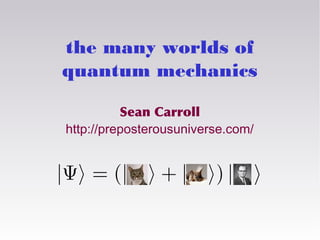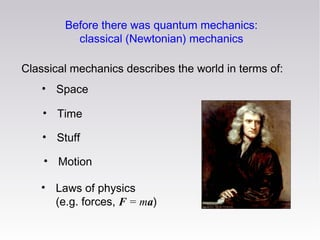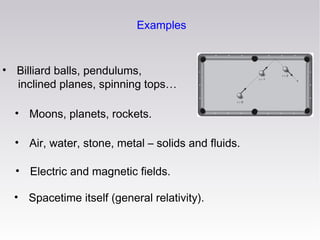Successfully reported this slideshow.

# The Many Worlds of Quantum Mechanics

59

Share×
1 of 36
1 of 36

# The Many Worlds of Quantum Mechanics

59

Share

A popular-level talk on quantum mechanics, concentrating on interference, entanglement, decoherence, and the Many-Worlds interpretation.

A popular-level talk on quantum mechanics, concentrating on interference, entanglement, decoherence, and the Many-Worlds interpretation.

## More Related Content

### Related Books

Free with a 14 day trial from Scribd

See all

### Related Audiobooks

Free with a 14 day trial from Scribd

See all

### The Many Worlds of Quantum Mechanics

1. 1. The Many Worlds of Quantum Mechanics Sean Carroll http://preposterousuniverse.com/
2. 2. Before there was quantum mechanics: classical (Newtonian) mechanics Classical mechanics describes the world in terms of: • Space • Time • Stuff • Motion • Laws of physics (e.g. forces, F = ma)
3. 3. Examples • Billiard balls, pendulums, inclined planes, spinning tops… • Moons, planets, rockets. • Air, water, stone, metal – solids and fluids. • Electric and magnetic fields. • Spacetime itself (general relativity).
4. 4. A clockwork universe If we knew the complete state of the universe at any one moment; and knew the laws of physics exactly; and had infinite computing power; we would know the state of the universe at every other moment. State of the universe = position and velocity of every particle/field “Laplace’s Demon”
5. 5. Quantum Mechanics: early hints, dawn of the 20th century Max Planck: blackbody radiation Albert Einstein: photons Henri Becquerel, Marie & Pierre Curie: radioactivity
6. 6. Niels Bohr: electrons in atoms can’t be located just anywhere; they only have certain allowed orbits
7. 7. 1920’s: quantum mechanics becomes a full-blown theory Werner Heisenberg: “matrix mechanics” Paul Dirac: Heisenberg’s and Schrödinger’s formalisms are equivalent Erwin Schrödinger: “wave mechanics” Newton’s Laws are replaced by the Schrödinger Equation for the quantum state:
8. 8. Quantum mechanics: the secret What we observe is much less than what actually exists.
9. 9. What is the “state” of a system? Classical mechanics: position and velocity. Quantum mechanics: the wave function. Position and velocity are what you can observe. But until you measure them, they don’t exist. Only the wave function does. The wave function tells you the probability of measuring different values of position or velocity.
10. 10. Electrons in atoms Cartoon: circling in orbits Reality: a static wave function
11. 11. Simple example: Miss Kitty, a two-state system (“qubit”) When we look for Miss Kitty, we only ever find her under the table, or on the sofa. Her wave function might give us a 50% chance of finding her under the table, 50% of finding her on the sofa.
12. 12. Classically: Miss Kitty is either under the table or on the sofa, we just don’t know which one. Quantum mechanics: she is actually in a superposition of both possibilities, until we look. Reason why: interference.
13. 13. Interference Imagine that we see Miss Kitty stop by either her food bowl or her scratching post on the way to the table or sofa. 50% 50% 50% 50% Either way, we find a 50/50 chance to see her on the table or the sofa at the end of her journey.
14. 14. But sometimes we don’t watch. She goes by either her food bowl or scratching post, we don’t know which. 0% 100% In that case, it turns out we always find her ending up on the sofa, never under the table! What’s going on?
15. 15. • For every possible observable outcome, the wave function has a value. wave function The wave function tells us the probability, but it’s not equal to the probability. position (or other observable) • Wave functions can be positive or negative *. Different contributions to the wave function can therefore either reinforce, or cancel each other out. • Probability of observing an outcome = (wave function) 2. * More precisely: wave functions are complex numbers, ψ = a + ib, and the probability is given by |ψ|2 = a2 + b2.
16. 16. 1926
17. 17. So if the wave function for Miss Kitty to be under the table is 0.71, the probability of finding her there is (0.71)2 = 0.50, or 50%. 0.71 0.71 But, crucially, if the wave function had been -0.71, we would have the same probability, since (-0.71)2 = 0.50 also. That’s what happened in our interference experiment.
18. 18. (0.71)2 = 0.5 (-0.71)2 = 0.5 (0.71)2 = 0.5 (0.71)2 = 0.5 ½(.71-.71)2 =0 ½(.71+.71)2 =1 Interference is at the heart of quantum mechanics.
19. 19. The Measurement Problem What actually happens when we observe properties of a quantum-mechanical system? Why should “measurement” or “observation” play a crucial role in a physical theory at all? Thus, “interpretations of quantum mechanics.”
20. 20. The Copenhagen (textbook) interpretation of quantum mechanics • The “quantum realm” is distinct from the macroscopic “classical realm” of observers. • Observations occur when the two realms interact. • Unobserved wave functions evolve smoothly, deterministically, via the Schrödinger equation. • Observed wave functions collapse instantly onto possible measurement outcomes. • After collapse, the new quantum state is concentrated on that outcome.
21. 21. In Copenhagen, our observation of Miss Kitty along her path collapsed her wave function onto “scratching post” or “food bowl,” eliminating future interference.
22. 22. A more complicated world Imagine we have both a cat and a dog: We can observe Ms. Kitty in one of two possible states: (on the sofa) or (under the table). We can also observe Mr. Dog in one of two possible states: (in the yard) or (in the doghouse). Classically, we describe the systems separately. In quantum mechanics, we describe them both at once.
23. 23. Entanglement There is one wave function for the combined cat+dog system. It has four possible “basis states”: sofa (sofa, yard) = sofa table (sofa, doghouse) = yard doghouse sofa (table, yard) = yard doghouse table yard doghouse table sofa (table, doghouse) = table yard doghouse
24. 24. Consider a state: ½(sofa, yard) + ½(sofa, doghouse) (cat, dog) = + ½(table, yard) + ½(table, doghouse) Each specific outcome has a probability (1/2)2 = 1/4 = 25% So Ms. Kitty has a total probability for (sofa) of 50%, likewise for (table); similarly for Mr. Dog. Knowing about Ms. Kitty tells us nothing about Mr. Dog, and vice-versa.
25. 25. Now instead, consider a state: (cat, dog) = 0.71(sofa, yard) + 0.71(table, doghouse) Each specific outcome has a probability (0.71)2 = 0.50 = 50% Again, Ms. Kitty has a total probability for (sofa) of 50%, likewise for (table); similarly for Mr. Dog. But now, if we observe Ms. Kitty under the table, we know Mr. Dog is in the yard with 100% probability – without even looking at him!
26. 26. sofa (sofa, yard) = yard doghouse sofa (table, yard) = sofa table (sofa, doghouse) = yard doghouse table yard doghouse table sofa (table, doghouse) = table yard doghouse Entanglement establishes correlations between different possible measurement outcomes.
27. 27. Decoherence Consider again an entangled state of Ms. Kitty and Mr. Dog: (cat, dog) = 0.71(sofa, yard) + 0.71(table, doghouse) But imagine we know nothing about Mr. Dog (and won’t). How do we describe the state of Ms. Kitty by herself? You might guess: (cat) = 0.71(sofa) + 0.71(table) But that turns out to be wrong.
28. 28. Entanglement can prevent interference With no entanglement, different contributions to Ms. Kitty’s path lead to the same final states (sofa or table): Since final states are the same, they can add or subtract (and thus interfere): via post: (cat) = 0.5(sofa) + 0.5(table) via bowl: (cat) = -0.5(sofa) + 0.5(table) total: (cat) = 0(sofa) + 1.0(table)
29. 29. Now imagine Ms. Kitty’s path becomes entangled with the state of Mr. Dog. (yard) (doghouse) Now the final states of the wave function are not the same (Mr. Dog and Ms. Kitty are entangled), and interference fails: via post: (cat, dog) = 0.5(sofa, yard) + 0.5(table, yard) via bowl: (cat, dog) = -0.5(sofa, doghouse) + 0.5(table, doghouse) total: (cat, dog) = 0.5(sofa, yard) + 0.5(table, yard) - 0.5(sofa, doghouse) + 0.5(table, doghouse)
30. 30. Upshot: when a quantum system becomes entangled with the outside world, different possibilities decohere and can no longer interfere with each other. If a system becomes entangled with a messy, permanent, external environment, its different possibilities will never interfere with each other, nor affect each other in any way. It’s as if they have become part of separate worlds.
31. 31. Many-Worlds Interpretation of Quantum Mechanics Hugh Everett, 1957 • There is no “classical realm.” Everything is quantum, including you, the observer. • Wave functions never “collapse.” Only smooth, deterministic evolution. • Apparent collapse due to entanglement/decoherence. • Unobserved possibilities – other “worlds” – still exist.
32. 32. Schrödinger’s Cat: Copenhagen version Ms. Kitty is in a superposition of (awake) and (asleep), then observed.
33. 33. Schrödinger’s Cat: Many-Worlds version Now we have Ms. Kitty, an observer, and an environment.
34. 34. Silly objections to Many-Worlds 1. That’s too many universes! The number of possible quantum states remains fixed. The wave function contains the same amount of information at any time. You’re really saying “I think there are too many quantum states.” 2. This can’t be tested! Many-worlds is just QM without a collapse postulate or hidden variables. It’s tested every time we observe interference. If you have an alternative with explicit collapses or hidden variables, we can test that!
35. 35. Reasonable questions for Many-Worlds 1. How do classical worlds emerge? The “preferred basis problem.” Roughly, the answer is because interactions are local in space, allowing some configurations to be robust and not others. 2. Why are probabilities given by the square of the wave function? For that matter, why are there probabilities at all? The theory is completely deterministic.
36. 36. “Despite the unrivaled empirical success of quantum theory, the very suggestion that it may be literally true as a description of nature is still greeted with cynicism, incomprehension, and even anger.” - David Deutsch

### Editor's Notes

• There is no such thing as “position” or “velocity”!
• Not just incomplete knowledge!